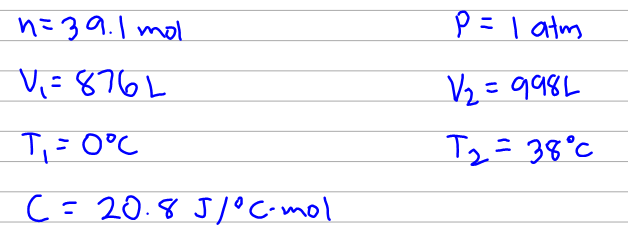# Problem: A balloon filled with 39.1 moles of helium has a volume of 876 L at 0.0°C and 1.00 atm pressure. The temperature of the balloon is increased to 38.0°C as it expands to a volume of 998 L, the pressure remaining constant. Calculate q, w, and ΔE for the helium in the balloon. (The molar heat capacity for helium gas is 20.8 J/°C • mol.)

###### FREE Expert Solution

This problem is pretty straight forward. We'll just need to use a bunch of equations and directly substitute our given values.

Before we work on our equations, let's just make sure that we have the correct units. The units for each type of parameter should be consistent.Here the units are all consistent so we don't have to convert anything.

100% (85 ratings)###### Problem Details

A balloon filled with 39.1 moles of helium has a volume of 876 L at 0.0°C and 1.00 atm pressure. The temperature of the balloon is increased to 38.0°C as it expands to a volume of 998 L, the pressure remaining constant. Calculate q, w, and ΔE for the helium in the balloon. (The molar heat capacity for helium gas is 20.8 J/°C • mol.)